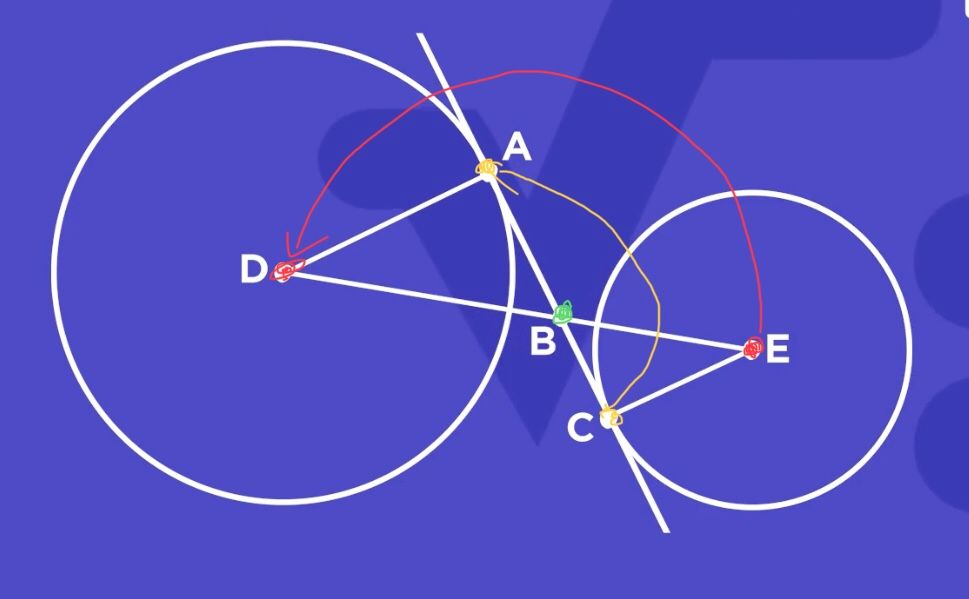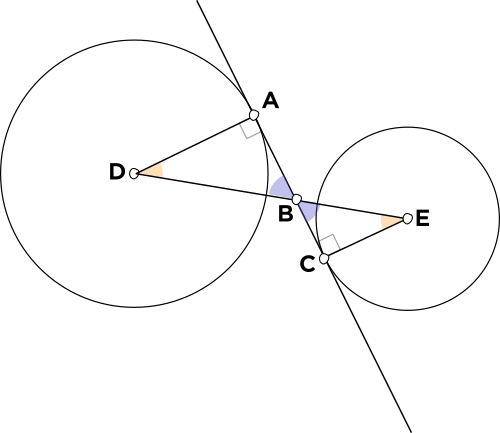# Order

• For the mini question, the correct answer, ii, says AB and BC.However, in this diagram I annotated, AB represents yellow->green and BC represents green->yellow instead.
Even though they are the same length, I think order is quite important and the answer should be changed to something like “AB and CB” or “BA and BC”.

Thanks• @RZ923 Thank you, this is a good suggestion!• @debbie Fixed• ???? huhThe answer choice originally read as "$$\textcolor{blue}{\overline{AB}}$$ and $$\textcolor{blue}{\overline{BC}},$$" and you can see the order of the vertices: in $$\overline{AB},$$ the first vertex, $$A,$$ is at the right angle and the second vertex, $$B,$$ is at the purple angle. In $$\overline{BC},$$ the first vertex, $$B,$$ is at the purple angle, and the second vertex, $$C,$$ is at the right angle.
You can also see from the similar triangles $$\bigtriangleup ABD$$ and $$\bigtriangleup CBE$$ that the side $$\overline{AB}$$ corresponds to the side $$\overline{CB}.$$